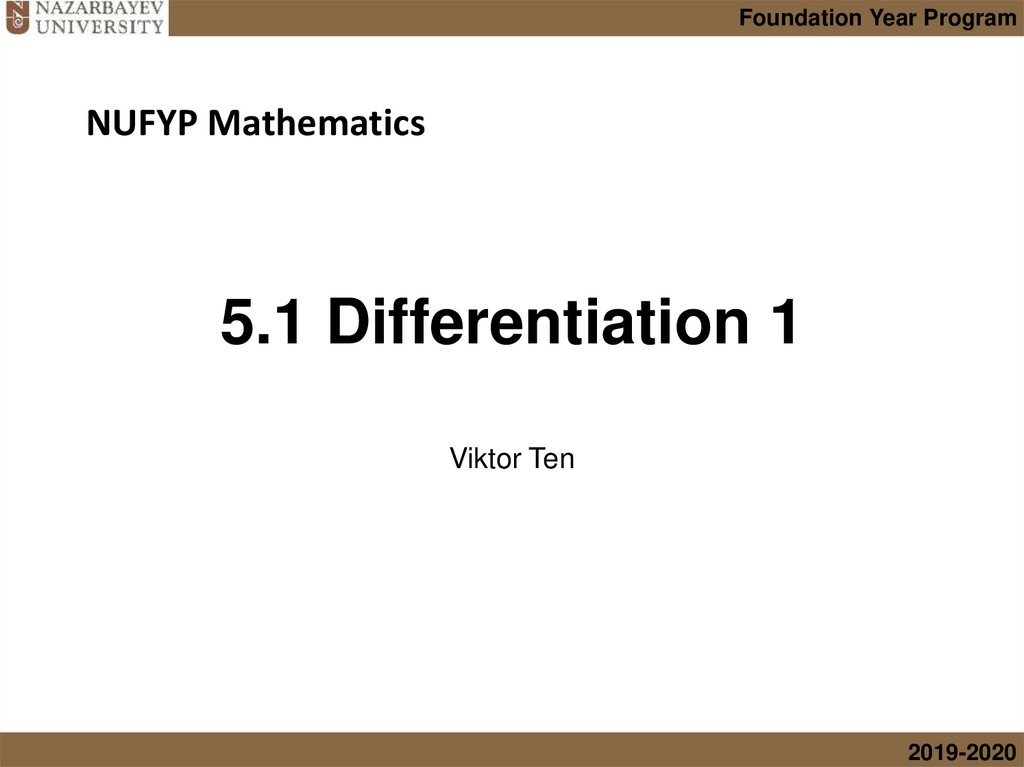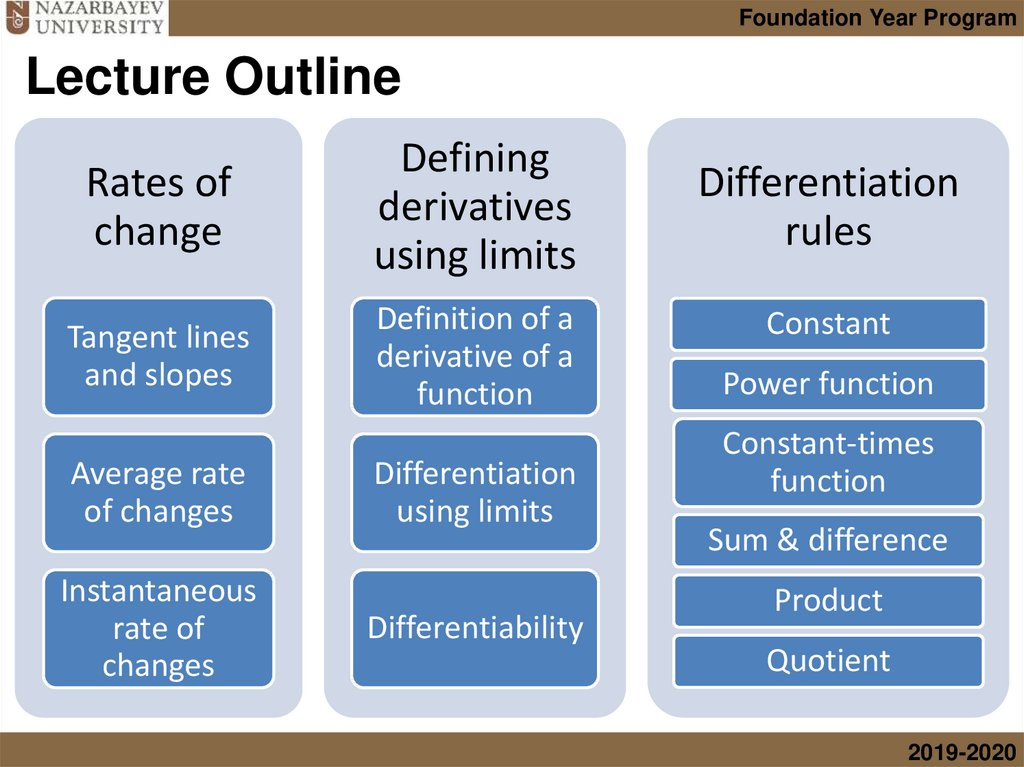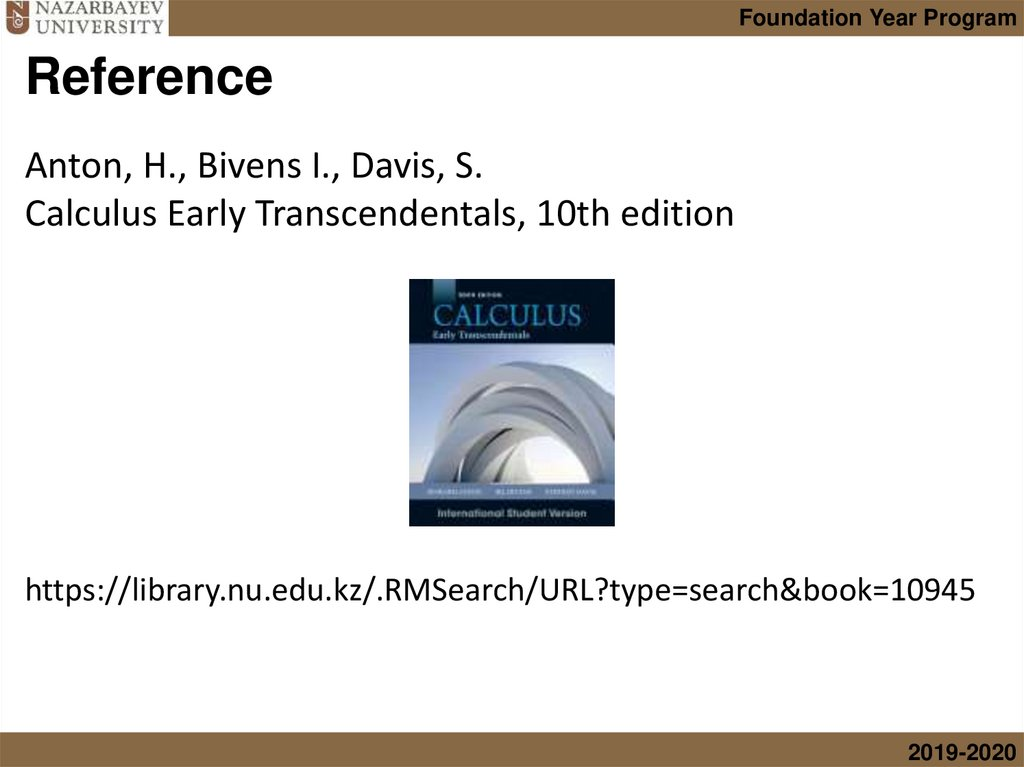# Differentiation. A “derivative”,

## 1. 5.1 Differentiation 1

Foundation Year Program
NUFYP Mathematics
5.1 Differentiation 1
Viktor Ten
2019-2020

## 2. Lecture Outline

Foundation Year Program
Lecture Outline
Rates of
change
Defining
derivatives
using limits
Tangent lines
and slopes
Definition of a
derivative of a
function
Average rate
of changes
Instantaneous
rate of
changes
Differentiation
using limits
Differentiability
Differentiation
rules
Constant
Power function
Constant-times
function
Sum & difference
Product
Quotient
2019-2020

## 3. Reference

Foundation Year Program
Reference
Anton, H., Bivens I., Davis, S.
Calculus Early Transcendentals, 10th edition
https://library.nu.edu.kz/.RMSearch/URL?type=search&book=10945
2019-2020

## 4. Introduction

Foundation Year Program
Introduction
Many real-world phenomena involve changing quantities:
• the speed of a rocket,
• the inflation of currency,
• the number of bacteria in a culture,
• the shock intensity of an earthquake,
• the voltage of an electrical signal, and so forth.
In this lecture we will develop the concept of a “derivative”,
which is the mathematical tool for studying the rate at
which one quantity changes relative to another.
2019-2020

## 5. Tangent lines

Foundation Year Program
Tangent lines
Consider a point